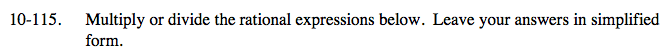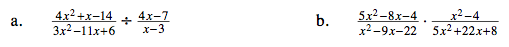### Home > AC > Chapter 10 > Lesson 10.3.2 > Problem10-115

10-115.Factor each expression.

$\frac{(4x-7)(x-2)}{(3x-2)(x-3)}\div\frac{(4x-7)}{(x-3)}$

Change the expression to multiplication.

$\frac{(4x-7)(x-2)}{(3x-2)(x-3)}\left(\frac{(x-3)}{(4x-7)}\right)$

Look for ones.

$\frac{(4x-7)}{(4x-7)}=\frac{(x-3)}{(x-3)}=1$

$\frac{(3x-2)}{(x-2)}$